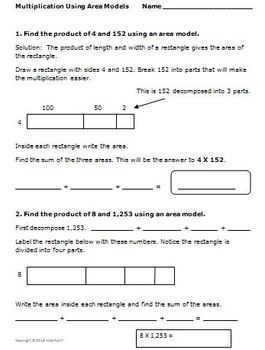# 4th Grade Math Multi-Digit Multiplication CCSS 4.NBT.54th
Subjects
Standards
Resource Type
Formats Included
• PDF
Pages
29 pages

### Description

Four strategies are taught to perform multi-digit multiplication. The resource includes multiplication of a one-digit number by a whole number of up to four digits as well as multiplication of two two-digit numbers.
The interactive notes provide for guided practice as students fill in blanks as the teacher presents the strategy. There are two of these examples for each activity along with two additional problems that students will attempt independently before the solution is discussed with teacher modeling. Each of the first three activities provides independent practice for the teacher to check for understanding.

Included in this product are:
1. Title Page
2. Detailed Teacher Notes
3. Student Handout - Interactive Notes and Practice Using Distributive Property To Perform Multi-Digit Multiplication
4. Student Handout - Interactive Notes and Practice Using Area Models To Perform Multi-Digit Multiplication
5. Student Handout - Interactive Notes and Practice Using Traditional Methods To Perform Multi-Digit Multiplication
6. Student Handout/Group Activity - Four Application Problems

This resource will guide the students through various strategies to perform multi-digit multiplication. Then they can choose the strategy they like best.

Common Core Math 4th Grade Geometry Activities Angles Shapes Symmetry (4.G)

Common Core Math 4th Grade Measurement (MD) Activities Area/Perimeter

Common Core Math 4th Grade Measurement Activities - Angles (4.MD.5,6,7)

Easter Math Common Core Activities Grade 4 (4.OA.3, 4.OA.4, 4.NF.2)

4th Grade Math Activities Division Strategies Multi-Digit Dividends CCSS 4.NBT.6

4th Grade Math - Equivalent Fractions - Compare Fractions - 4.NF.1, 4.NF.2

4th Grade Math - Multiplication/Division, Fractions, 2-D Shapes, Symmetry

Created by Hilda Ratliff
Total Pages
29 pages
Included
Teaching Duration
N/A
Report this Resource to TpT
Reported resources will be reviewed by our team. Report this resource to let us know if this resource violates TpT’s content guidelines.

### Standards

to see state-specific standards (only available in the US).
Multiply a whole number of up to four digits by a one-digit whole number, and multiply two two-digit numbers, using strategies based on place value and the properties of operations. Illustrate and explain the calculation by using equations, rectangular arrays, and/or area models.